# 月度存档: 十二月 2016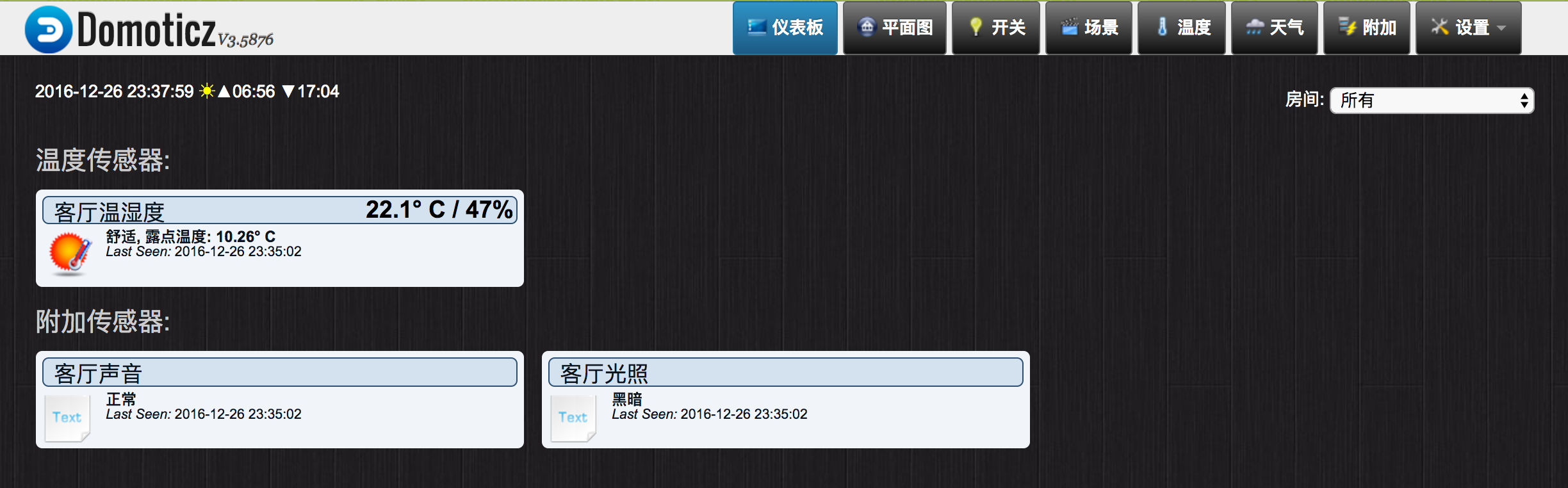## 准备工作

• DSM 6
• Domoticz v3.5876

## 创建设备及虚拟传感器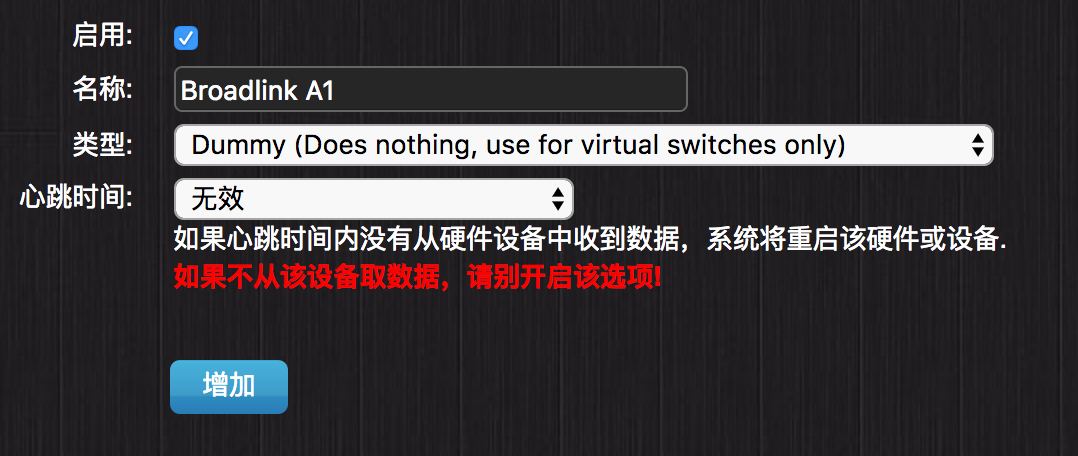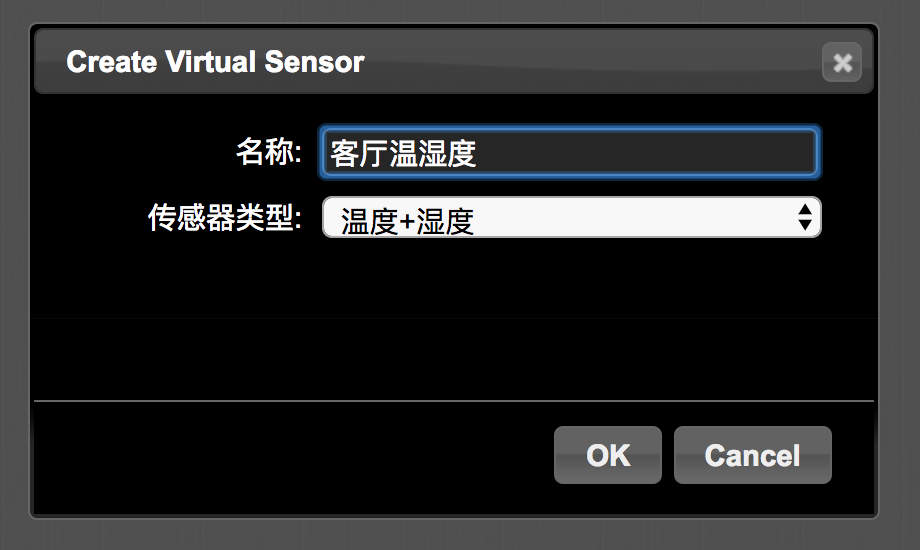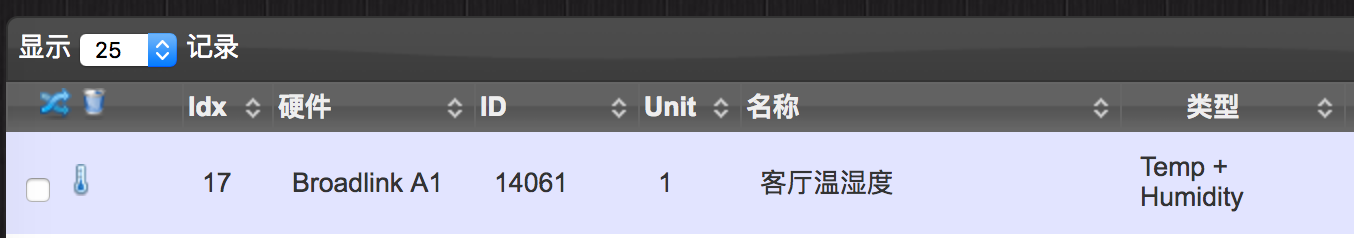## 在 DSM 上部署更新脚本

```#!/usr/bin/python
# -*- encoding: utf-8 -*-

DOMOTICZ_IP = '1.1.1.1' # 修改为 Domoticz 服务器 IP
DOMOTICZ_PORT = 8084 # 修改为 Domoticz 服务端口

DEV_HUM_TEMP_IDX = 1 # 修改为“温度+湿度”虚拟传感器Idx
DEV_NOISE_IDX = 2 # 修改为“声音”虚拟传感器Idx
DEV_LIGHT_IDX = 3 #修改为“光照”虚拟传感器Idx

import requests
import urllib

a1.auth()
data = a1.check_sensors_raw()

hum = data['humidity']
hum_stat = 0
if 45 < = hum <= 60:
hum_stat = 1
elif hum < 40:
hum_stat = 2
elif hum > 70:
hum_stat = 3

# update temp and hum
url = "http://%s:%d/json.htm?type=command¶m=udevice&idx=%d&nvalue=0&svalue=%0.1f;%0.1f;%d" % (DOMOTICZ_IP, DOMOTICZ_PORT, DEV_HUM_TEMP_IDX, data['temperature'], hum, hum_stat)
requests.get(url)

# update noise
db = '未知'
noise = data['noise']
if noise == 0:
db = '寂静'
elif noise == 1:
db = '正常'
elif noise == 2:
db = '吵闹'
db = urllib.quote(db)
url = "http://%s:%d/json.htm?type=command¶m=udevice&idx=%d&nvalue=0&svalue=%s" % (DOMOTICZ_IP, DOMOTICZ_PORT, DEV_NOISE_IDX, db)
requests.get(url)

# update light
light = data['light']
lux = '未知'
if light == 0:
lux = '黑暗'
elif light == 1:
lux = '昏暗'
elif light == 2:
lux = '正常'
elif light == 3:
lux = '明亮'
lux = urllib.quote(lux)
url = "http://%s:%d/json.htm?type=command¶m=udevice&idx=%d&nvalue=0&svalue=%s" % (DOMOTICZ_IP, DOMOTICZ_PORT, DEV_LIGHT_IDX, lux)
requests.get(url)
```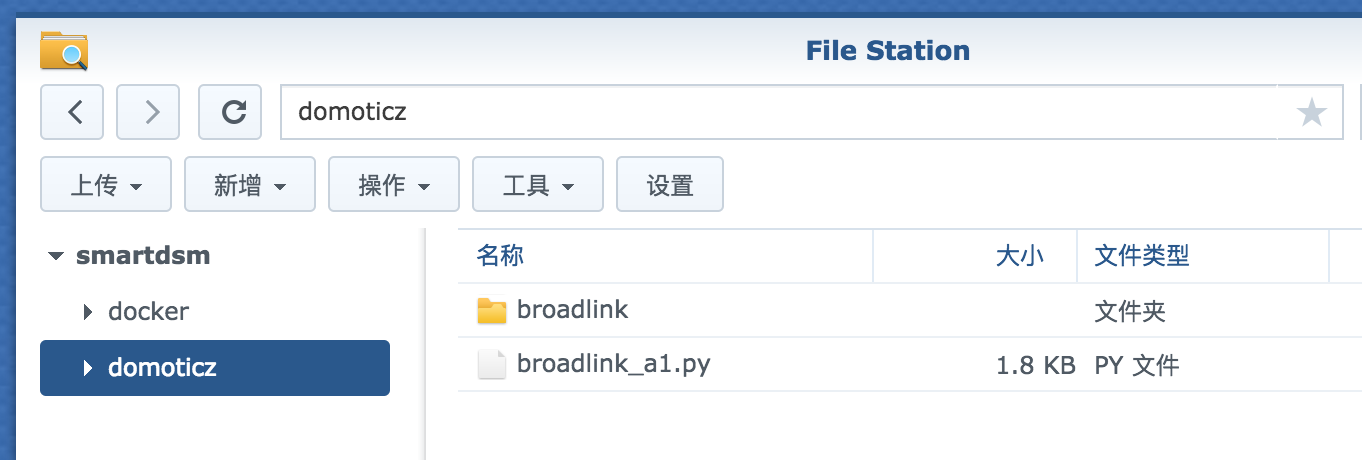## 添加计划任务定时更新 A1 数据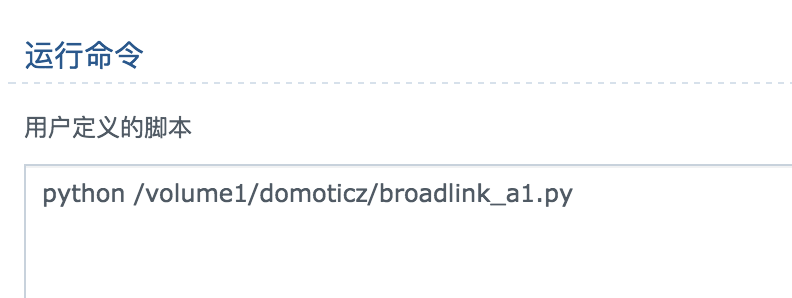— EOF —